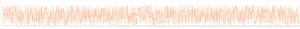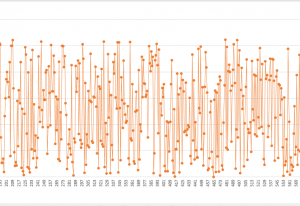## RSA illustration with not-so-small numbers – part 2

Let’s have a closer look at the encryption. During the communication, what’s been exposed are:

Alice’s public key (n=2627, e=13) , and the encrypted message.

For anyone who’s entered the world of modern cryptography from the old age, it’s tempting to try to decrypt the encrypted message using the encrypting key, the public key.

For these people, I have the below chart that shows the mapping between the plain text and the encrypted data:x-axis is the plain-text data (sorted from 1 to 2627) and y-axis is the encrypted data(from 0 to 2626). I did the calculation using this line of script:

~$for i in seq 1 2627; do echo "$i^13 %2627" | bc; done > /tmp/encryption.mapping

Below is part of this chart zoomed-in:So you know the encrypted data, let’s say 2144, and you know the public key (n=2627, e=13). How do you find the number x such that x^13 % 2627 = 2144.

You cannot unless you compute everyone possible 1<x<2627 and then find the correct one. That’s brutal force. This is one of the basic assumption behind the security of RSA: There’s no efficient way to find x. This is called the discrete logarithm problem.

In real world scenarios, the 2 prime numbers will be so large that brutal force is simple impractical.

Then to decrypt the message, one would need the private key. The private key is the modular inverse of phi(n). However, in order to get phi(n), he has to know the factors that form n. And factoring large number is mathematically hard. That is the other assumption behind the security of RSA: There’s no efficient way to factor a large number.

As you will see in other places, these 2 assumptions are the corner stones of modern cryptography.

## RSA illustration with not-so-small numbers

Modern cryptography is difficult to understand without illustrations. One of the reason is, modern cryptography involves very large numbers that easily exceed the capacity of a standard calculator, let alone human comprehension. There are some illustrations out there using small numbers. The problem is, the numbers are too small to be convincing. So I’d like to try some no-so-small numbers here. Most of the necessary calculations can be done with GNU bc, so you can try yourself on just any GNU Linux distribution.

Let’s say Bob wants to send the below number to Alice (and make sure only Alice can decrypt the message):

520

Here’s what Alice will do first:

1. Pick up two distinct prime numbers. The numbers should be sufficiently large so that brutal force is difficult. Here we choose p=37 and q=71.
2. Calculating n=pq=37*71=2627.
3. Calculating the n‘s totient function: phi(n)=(p-1)*(q-1)=2520.
4. Pick a number e between 1 and phi(n) that is co-prime with phi(n). Here we choose 13.
5. Find number d so that e*d mod (phi(n)) =1. Here we choose 1357. This step cannot be done with bc. Intead, you can try this online calculator. Just put “modinv(13,2520)” in the text field and then press “go” you’ll get the result.

Now Alice has a public key (n=2627, e=13) and a private key (n=2627, d=1357). She can simply distribute her public key to everyone, including Bob.

Now for Bob to encrypt the message 520 to Alice, he has to encrypt the message using Alice’s public key:

520^13 % 2627 = 2235

Now Alice received this number 2235 from Bob. In order to decrypt this message, she do the following calculation:

2235^1357 % 2627 = 520

Actually, here Bob can encrypt just any number that is less than or equal to n in this way.

Bob:

1^13 % 2627 = 1

Alice:

1^1357 % 2627 = 1

Bob:

2^13 % 2627 = 311

Alice:

311^1357 % 2627 = 2

Bob:

3^13 % 2627 = 2361

Alice:

2361^1357 % 2627 = 3

Bob:

4^13 % 2627 = 2149

Alice:

2149^1357 % 2627=4

Bob:

137^13 % 2627 = 2431

Alice:

2431^1357 % 2627 = 137

If his message is large, then he has to split his message into chunks that are smaller than n and encrypt them one by one.

Note that this only illustrates how Bob can send secrete messages to Alice. If Alice wants to send secrete messages to Bob then she has to have Bob do the same first:

1. Pick up 2 sufficiently large prime numbers;
2. Get the product of these 2 prime numbers – This is part of the keys;
3. Get the totient of this product;
4. Pick a number that is co-prime with this totient but smaller – This combined with the product is the public key;
5. Find the number that is the multiply modular inverse of this number – This combined with the product is the private key;

Then Bob sends his public key to Alice and Alice can encrypt the messages using Bob’s public key. Upon receiving the messages, Bob can decrypt the messages using his private key.